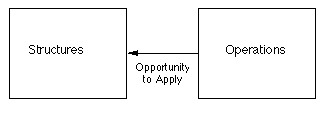Computer-based Simulation Modelling for Anthropologists Michael D. Fischer

Simulation Home

Introduction

 What is a computer simulation?

 Despite my earlier claims, this is, unfortunately, not such an easy question to answer from the literature. As with many terms in the literature, computer simulation is not so much defined as described.  Johnson 'defines' a computer simulation as

 ...a computer program that defines the variables of a system, the range of values those variables may take on, and their interrelations in enough detail for the system to be set in motion to generate some output. The main function of a computer simulations is to explore the properties and implications of a system that is too complex for logical or mathematical analysis... A computer simulation generally has an ad hoc or “home-made” quality that makes it less rigorous than a mathematical model  (1978:186-7)

 Nardi offers as a description:

 A computer simulation model ... [provides] the investigator with a simplified analogy ... for the purpose of better analysing and understanding ... [some] phenomenon. ... it focuses on conducting experiments on a computer in which mathematical or logical operations describing the behaviour of a system over time are of primary importance. ... Its very purpose, in fact, is the analysis of change over time. ... Computer simulation is a powerful technique, capable of handling large numbers of variables representing complex systems and of simulating the operation of these variables over many cycles.  (1980: 38)

 Davies and O'Keefe, in a programming guide for simulation, suggest:

 When the word [simulation] is used by computer scientists, statisticians, and management scientists, they normally refer to the construction of an abstract model representing some system in the real world. The simulation describes the pertinent aspects of the system as a series of equations and relationships, normally embedded in a computer program. (1989:1)

 These descriptions of simulation might be adequate for many purposes, but it is worth a closer look at a more structural definition of simulation in context of how anthropologists have used them and might use them, if for no other reason than to provide a basis for designing simulations and interpreting the results, a subject treated with extreme coyness in the literature.

 Specifying the structure of a simulation is very much like specifying any problem for computer treatment: we have to visualise what we want to get out of a simulation, and devise a structure that will fulfil this goal. Most descriptions of simulation, especially those by anthropologists, suggest that a simulation model is:

 a) used to model systems too complex to model with ordinary analytic models b) comprised of logical or mathematical operations on variables c) able to represent a large number of variables and operations d) holistic in orientation, often used to model  aspects of entire systems e) oriented towards representing processes f) oriented towards exploration and experimentation

 Items an and b suggest that although simulations are composed of analytic models, simulations are not necessarily analytic models (Johnson 1978:186-7). In other words, simulations can be informal models defined partly in terms of formal models 18. Items c and d reflect a view that simulations are suitable for modelling complex large scale systems as well as simple small scale systems (Johnson 1978:187; Nardi 1980:38), and are thus 'capable of greater realism' (Johnson 1978:187). Item e expresses a pervasive attitude that simulations model processes. It is more accurate to say that simulations produce their results from one or more processes. These processes may be used as part of a model of systemic processes, or they may simply be artefacts of the simulation 19. Most simulations have been used to model processes, but incorporate both relevant and artefactual processes. Item f indicates a pre-analytic bias in the use of simulation; simulations are oriented towards producing model data for analysis, not solutions (Buchler et al 1986: 112), although the computer implementation of a simulation model may include analytic models which apply to the model generated data, and simulations may be used in some cases when no other solution is plausible or possible (Dyke 1981:204).

 These properties suggest a number of possible structures, but are not complete. What distinguishes a simulation model from any other model forms is not so much the type of model, but what we do with the model. In the case of simulations we are interested in the behaviour of a model; instances of application of a model. Simulations do not have solutions in the  conventional sense. The most appropriate purpose of a simulation is to generate data, representing the interaction of the models under simulation. The value and purpose of a simulation follows from what is done with this model data (Dyke 1981:204).

 Extending this, I propose a more general structure for computer simulations.  Abstractly, a simulation model consists of at least one structure, at least one operation which might act on the structure(s), and at least one opportunity to apply operation(s) to structure(s) (Figure 1). It is the applications of one or more models to create one or more instances. An operation may or may not be based on an analytic model; it can be quite ad hoc. A simulation is at least one instance of an application of operation to structure. This definition does not differentiate between the application of analytic models, such as a discriminant function derived from social data, and less formal models, such as those derived from so- called qualitative analysis of social data.Figure 1.  Abstract model of simulation